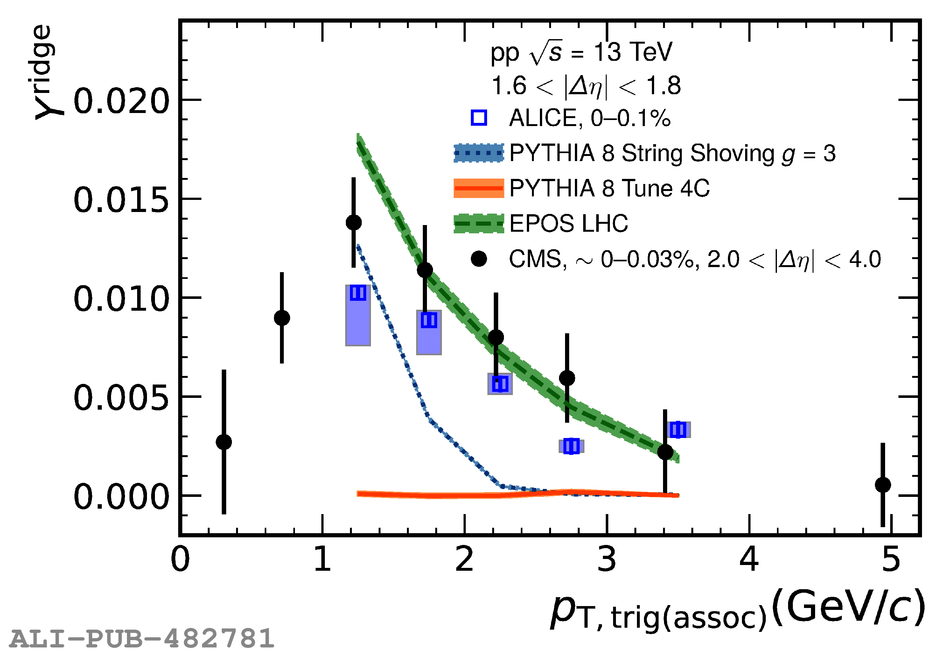# Figure 3

 < p> Fully corrected near-side ridge yield as a function transverse momentum. The open blue boxes denote the measurement by ALICE. The statistical and systematic uncertainties are shown as vertical bars and boxes, respectively. The CMS measurement~ is represented by filled circles and extends down to lower $\pt$ due to the larger $\Delta\eta$ acceptance. The three lines show model predictions from $\pythiam$ (blue dotted line), $\pythiashoving$ (orange line), and $\epos$ (green dashed line).< /p>